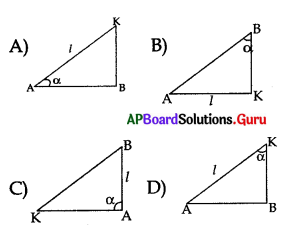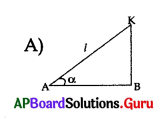Practice the AP 10th Class Maths Bits with Answers Chapter 12 Applications of Trigonometry on a regular basis so that you can attempt exams with utmost confidence.

## AP State Syllabus 10th Class Maths Bits 12th Lesson Applications of Trigonometry with Answers

Question 1.
If the angle of elevation of sun is 45°, then the length of the shadow of a 12 m
high tree is …………………
A) 12 √3 m
B) 16 m
C) 12 m
D) $$\frac{12}{\sqrt{3}}$$ m
C) 12 m

Question 2.
Observe the figure. Length of the ladder = ……………………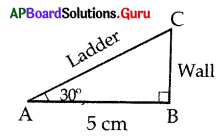A) 5 cm
B) 10 √3 cm.
C) 20 m.
D) 2.5 m
B) 10 √3 cm.Question 3.
The angle of depression from the top of a tower 12 m height, at a point on the ground is 30°. The distance of the point from the top is ……………….
A) 12 m
B) 12 √3 m
C) 7. 5 m
D) 6 m
A) 12 m

Question 4.
If the shadow of the tree is $$\frac{1}{\sqrt{3}}$$ times the height of the tree, then the angle of elevation of the sun is …………………..
A) 45°
B) 30°
C) 70°
D) 60°
D) 60°

Question 5.
The angle of elevation of a jet plane from a point A on the ground is 60°. After a flight of 15 seconds, the angle of elevation changes to 30°. If the jet plane is flying at a constant height of 1500 √3 meter, then the speed of the plane is ……………….. m/sec.
A) 200
B) 300
C) 400
D) 100
A) 200

Question 6.
The angle of elevation of top of a tower at a distance of 500 m from the foot is 30°. Then the height of the tower is ………………..
A) $$\frac{500}{\sqrt{3}}$$
B) 500 √3
C) $$\frac{3}{500}$$
D) $$\frac{\sqrt{3}}{500}$$
A) $$\frac{500}{\sqrt{3}}$$

Question 7.
If altitude of the sun is 60°. Then the height of the tower which is casting a shadow of 30 m. is ………………… m.
A) 61.69
B) 51.69
C) 51.96
D) 41.69
C) 51.96

Question 8.
If a 6m height pole casts a shadow 2√3 m long on the ground, then the sun’s angle of elevation is
A) 60°
B) 45°
C) 30°
D) 90°
A) 60°

Question 9.
From a point 30m from the foot of a tower, the angle of elevation of the top is 30°. Then the height of the tower is
A) 10 m
B) 10√3 m
C) 15 m
D) 19 m
B) 10√3 mQuestion 10.
From a ship masthead 150 ft high, the angle of depression of a boat is observed to 45°. Its distance from the ship is
A) 150 ft
B) 75 ft
C) 150√3 ft
D) $$\frac{150}{\sqrt{3}}$$ ft
A) 150 ft

Question 11.
A ladder of 19m is leaning to a wall making an angle of 60° with the ground. The distance from the foot of the wall to the foot of the ladder is
A) 18m
B) 19m
C) 9m
D) 9.5m
D) 9.5m

Question 12.
A boy observes the top of an electronic pole at an angle of elevation of 60°, when the observation point is 8m away from the foot of the pole. Then the height of the pole is
A) 6√3 m
B) 8√3m
C) 10√3m
D) 16√3 m
B) 8√3m

Question 13.
Raj ender observes a person standing on the ground from a helicopter at an angle of depression 45°. If the helicopter flies at a height of 50 m from ground, then the distance of the person from Raj ender is
A) 25 √2 m
B) 50 √2 m
C) 75 √2 m
D) None
B) 50 √2 m

Question 14.
The angle of elevation of the top of the cliff from a point 300m from its foot is 60°. Then the height of the cliff is
A) 200 √3 m
B) $$\frac{200}{\sqrt{3}}$$ m
C) 300√3 m
D) $$\frac{300}{\sqrt{3}}$$ m
C) 300√3 m

Question 15.
The angle of elevation of the top of a tower from the top and foot of a pole of height 10m are 30° and 60°. Then the height of the tower is
A) 5 m
B) 10 m
C) 15 m
D) 20 m
C) 15 m

Question 16.
A 1.5m tall boy casts 2m shadow. At the same instance, a lamppost casts a shadow of 10 m. Then the height of the lamppost is
A) 6.5 m
B) 7.5 m
C) 8.5 m
D) None
B) 7.5 m

Question 17.
The ratio of the length of a rod and its shadow is 1 : √3 Then the angle of elevation of the sun is …………………..
A) 45°
B) 30°
C) 75°
D) 90°
B) 30°Question 18.
A ladder ‘x’ meters long is laid against a wall making an angle ‘θ ‘ with the ground. If we want to directly find the distance between the foot of the ladder and the foot of the wall, which trigonometrical ratio should be considered ?
A) sin θ
B) cos θ
C) tan θ
D) cot θ
B) cos θ

Question 19.
Two persons A and B observe the top of a pole at an angle of elevation α and β respectively. If α > β, then ………………..
A) A is nearer to the pole than B.
B) B is nearer to the pole than A.
C) A, B are at the same distance from the pole.
D) Can’t compare their distances
C) A, B are at the same distance from the pole.

Question 20.
The angle of elevation of the top of a tower from a point at a distance 40 m from the foot of the tower is 45°. Then the height of the tower is
A) 20 m
B) 40 m
C) 40 √2 m
D) 40 √3 m
B) 40 m

Question 21.
The length of the shadow of a tower is equal to its heights, then the angle of elevation of the sun is
A) 90°
B) 70°
C) 60°
D) 45°
D) 45°

Question 22.
What is the angle of elevation of the top of a temple of height 10 m. At a point whose distance from the base of the tower is 10 √3 m
A) 30°
B) 60°
C) 45°
D) 90°
A) 30°

Question 23.
From the top of a 10 m height tree, the angle of depression of a point on the ground is 30°. Then the distance of the point from the foot of the tree is
A) 10m
B) 10 √3 m
C) $$\frac{10}{\sqrt{3}}$$ m
D) 5 √3 m
B) 10 √3 m

Question 24.
The angle of elevation of the sun is 45° then the length of the shadow of a 12 m high tree is ………………
A) 12√3
B) 16
C) 12
D) 16 √3
C) 12Question 25.
The length of the shadow is of 5 m height tree whose angle of elevation of the sun is 30° is
A) 5 m
B) √3 m
C) 5 √3 m
D) 10 m
C) 5 √3 m

Question 26.
A man observed the top point of a flag staff with an angle of elevation of 45° standing at a distance away from the bottom of the staff . Find the distance between the man and the staff, if the height of the staff is 50 m.
A) 50 m
B) 60 m
C) 30 m
D) 22.5 m
A) 50 m

Question 27.
A man observed the top point of a chimney with angle of elevation 60° standing at a distance 90 m. away from the bottom of the chimney. Then the height of the chimney is …………………..
A) $$\frac{90}{\sqrt{3}}$$ m
B) $$\frac{45}{\sqrt{3}}$$ m
C) 90 √3 m
D) 45 √3 m
C) 90 √3 m

Question 28.
A man observed the top of a hill with an angle of elevation of 50° standing at a distance away from the bottom of the hill. If another man stands on the hill, at what angle of depression did he see the first man ?
A) 60°
B) 50°
C) 70°
D) 80°
B) 50°

Question 29.
When the sun rays are falling on the earth at an inclination of 45 0 the shadow of a man is 1.5 metres. Man’s height is …………………
A) 1.2 m
B) 1 m
C) 1.5 m
D) 1.732 m
C) 1.5 m

Question 30.
Two towers of heights X and Y. A person standing in between X and Y, he observed the top of the tower at an angles of elevation 30° and 60°. Then the value of X : Y is ……………….
A) 3 : 1
B) 1 : √3
C) √3 : 1
D) 1 : 3
D) 1 : 3

Question 31.
The length of the shadow of wall of height 8 m is 5 m. At the same time the tower of height is 50 m its length of the shadow is
A) 200 m
B) 80 m
C) 40 m
D) 20 m
B) 80 mQuestion 32.
If the angle of elevation of the sun is 60° then the ratio of height of a tree with its shadow is
A) 1 : 1
B) 1 : √3
C) √3 : 1
D) 1 : 3
C) √3 : 1

Question 33.
The angle of elevation of a top of the tower from a point 120 m. from its foot is 45°. How much the tower is to be raised when the elevation is to be 60° at the same point ?
A) 120 √3 m
B) 120 m
C) 120(√3 + 1) m
D) 120(√3 – 1) m
D) 120(√3 – 1) m

Question 34.
A ladder 19 m leaning to wall at 60° with the ground, the distance from foot to the wall is
A) 18 m
B) 19m
C) 9 m
D) 9.5 m
D) 9.5 m

Question 35.
From a ship mast head 150 m high the angle of depression of a boat is observed to be 60° its distance from the ship is ………………..
A) 75.5 m
B) 86.55 m
C) 92.55 m
D) 120.5 m
B) 86.55 m

Question 36.
a) Srinu said “Angle of elevation is equal to the angle of depression.”
b) Srikanth said “Angle of depression is equal to the angle of elevation.”
A) Only A is True
B) Only B is True
C) Both A and B are True
D) Both A and B are False
C) Both A and B are True

Question 37.
Sin (2 × 30°) = 2 Sin 30° statement is
A) True
B) False
C) Can’t say
D) Not defined
B) FalseQuestion 38.
Sin a = $$\frac{x}{y}$$ ; cos a = $$\frac{\mathrm{a}}{\mathrm{b}}$$ then $$\frac{\text { bx }}{\text { ay }}$$ represent
A) Tan α
B) cot α
C) sec α
D) cosec α
A) Tan α

Question 39.
The length of shadow of a tree of 8 m long. When the son’s angle of elevation is 45° is ……………… m.
A) $$\frac{8}{\sqrt{3}}$$
B) 8 √3
C) 8
D) 16 √3
C) 8

Question 40.
The angle of elevation of the top of a tower from a point on the ground which is 30 m away from the foot of the tower is 30°. Then the height of the tower is
A) 10 √3 m
B) $$\frac{10}{\sqrt{3}}$$ m
C) $$\frac{\sqrt{3}}{10}$$
D) $$\frac{10}{2 \sqrt{3}}$$ m
A) 10 √3 m

Question 41.
In the adjacent figure ‘θ’ is called as angle of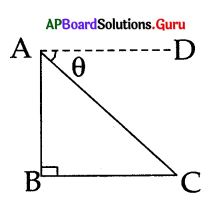A) elevation
B) distance
C) depression
D) length
C) depression

Question 42.
The height of the tower, in the given figure.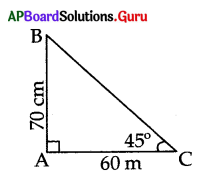A) 45 m
B) 60 m
C) 50 m
D) 90 m
B) 60 m

Question 43.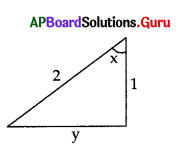a) Ravi said ‘y = √3’
b) Ramu said ‘y = V3 ‘
c) Kiran said ‘ y = $$\frac {1}{2}$$ ‘
Do you agree with whom ? (Or)
With whom do you agree ? ………………..
A) Ravi
B) Kiran
C) Ramu
D) All of them